Author: Oscar Cronquist Article last updated on February 05, 2019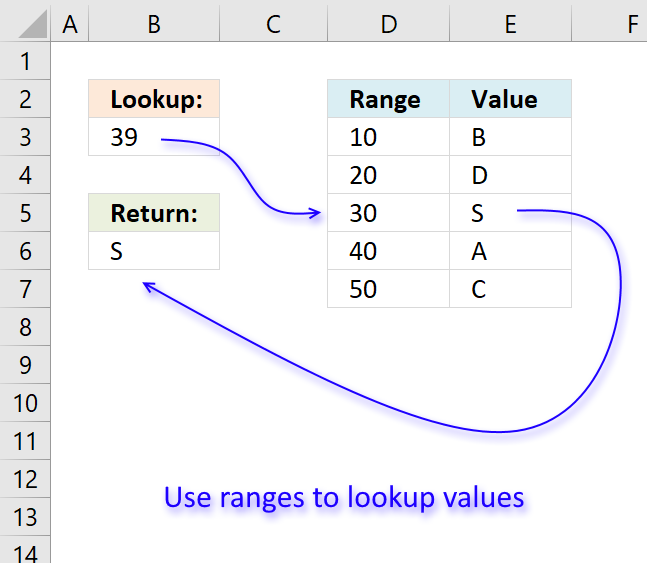In this article, I will demonstrate four different formulas that allow you to lookup a value that is to be found in a given range and return the corresponding value on the same row. If you need to return multiple values because the ranges overlap then read this article: Return multiple values if in range.

They all have their pros and cons and I will discuss those in great detail, they can be applied to not only numerical ranges but also text ranges and date ranges as well.

I have made a video that explains the LOOKUP function in context to this article, if you are interested.

There is a file for you to get, at the end of this article, which contains all the formula examples in a worksheet each.

You can use the techniques described in this article to calculate discount percentages based on price intervals or linear results based on the lookup value.

Check out the LOOKUP category to find more interesting articles.

The following table shows the differences between the formulas presented in this article.

 Formula Range sorted? Array formula Get value from any column? Two range columns? LOOKUP Yes No Yes No INDEX + SUMPRODUCT + ROW No No Yes Yes VLOOKUP Yes No No No INDEX + MATCH Yes No Yes No

Some formulas require you to have the lookup range sorted to function properly, the INDEX+SUMPRODUCT+ROW alternative is the only way to go if you can't sort the values.

The disadvantage with the INDEX+SUMPRODUCT+ROW formula is that you need start and end values, the other formulas use the start values also as end range values.

The VLOOKUP function can only search the leftmost column, you must rearrange your table to meet this condition if you are going to use the VLOOKUP function.

### LOOKUP function

To better demonstrate the LOOKUP function I am going to answer the following question.

Hi,

What type of formula could be used if you weren't using a date range and your data was not concatenated?

ie: Input Value 1.78 should return a Value of B as it is between the values in Range1 and Range2

Range1 Range2 Value
1.33 1.66 A
1.67 1.99 B
2.00 2.33 C

The next image shows the table in greater detail.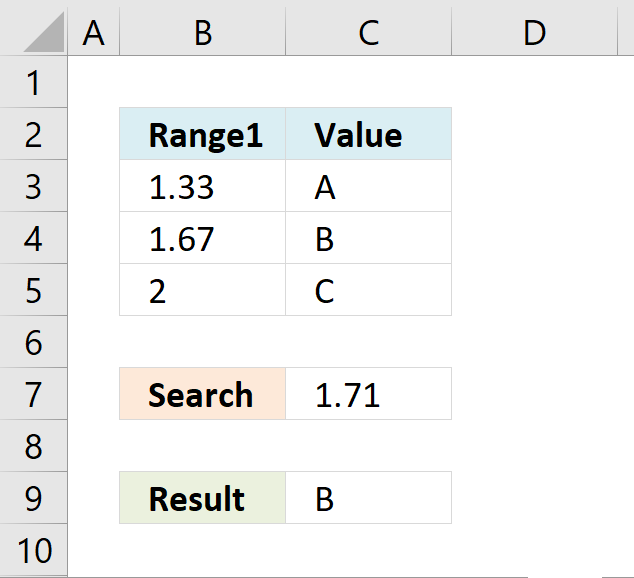The picture above shows data in cell range B3:C5, the search value is in C7 and the result is in C9.

Cell range B3:B5 must be sorted in ascending order for the LOOKUP function to work properly.

Ascending order means values are sorted from the smallest to the largest value. Example: 1,5,8,11.

If an exact match is not found the largest value is returned as long as it is smaller than the lookup value.

The LOOKUP function then returns a value in a column on the same row.

The formula in cell C9:

=LOOKUP(C8,B4:B6,C4:C6)

Example, Search value 1.71 has no exact match, the largest value that is smaller than 1.71 is 1.67. The returning value is found in column C on the same row as 1.67, in this case, B.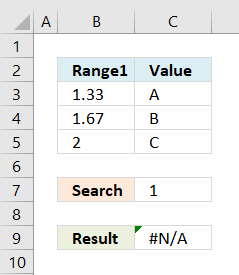If the search value is smaller than the smallest value in the lookup range the function returns #N/A meaning Not Available or does not exist.

Example in the picture to the right, search value is 1 in the and the LOOKUP function returns #N/A.

To solve this problem simply add another number, for example 0. Cell range B3:B6 would then contain 0, 1.33, 1.67, 2.

A search value greater than the largest value in the lookup range matches the largest value. Example in above picture, search value is 3 and the returning value is C.

Watch video below to see how the LOOKUP function works:

How to use the LOOKUP function

Finds a value in a sorted cell range and returns a value on the same row.

#### Tip! - You can quickly sort a cell range, follow these steps:

1. Press with right mouse button on on a cell in the cell range you want to sort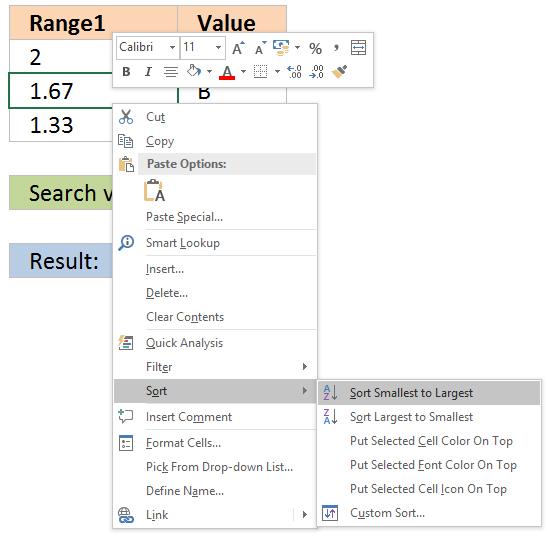2. Hover with mouse cursor over Sort
3. Press with mouse on "Sort Smallest to Largest"

### INDEX + SUMPRODUCT + ROW

The following formula is slightly larger but you don't need to sort cell range B4:B6.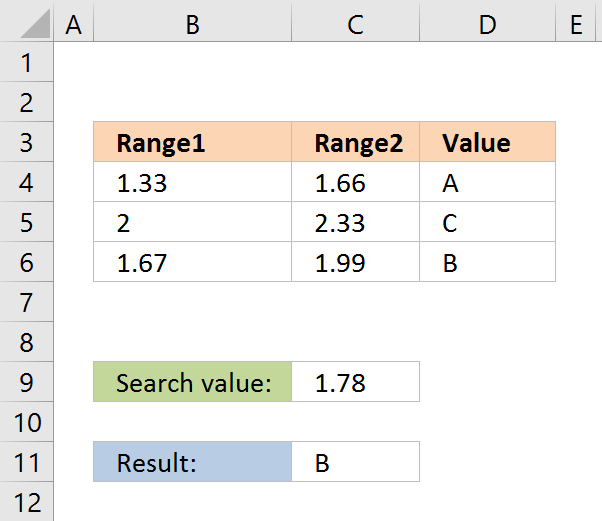The formula in cell C11:

=INDEX(D4:D6, SUMPRODUCT(--(\$D\$8<=C4:C6), --(\$D\$8>=B4:B6), ROW(A1:A3)))

The ranges don't need to be sorted however you need a start (Range1) and an end value (Range2).

#### Explaining formula in cell C11

You can easily follow along, go to tab "Formulas" and press with left mouse button on "Evaluate Formula" button. Press with left mouse button on "Evaluate" button to move to next step.

Step 1 - Calculate first condition

The bolded part is the the logical expression I am going to explain in this step.

=INDEX(D4:D6, SUMPRODUCT(--(\$D\$8<=C4:C6), --(\$D\$8>=B4:B6), ROW(A1:A3)))

Logical operators

= equal sign
> less than sign
< greater than sign

The gretaer than sign combined with the equal sign <= means if value in cell D8 is smaller than or equal to the values in cell range C4:C6.

--(\$D\$8<=C4:C6)

becomes

--(1,78<={1,66;1,99;2,33})

becomes

--{1,78<=1,66; 1,78<=1,99; 1,78<=2,33})

becomes

--({FALSE;TRUE;TRUE})

and returns {0;1;1}.

The double minus signs convert the boolean value TRUE or FALSE to the corresponding number 1 or 0 (zero).

Step 2 - Calculate second criterion

=INDEX(D4:D6, SUMPRODUCT(--(\$D\$8<=C4:C6), --(\$D\$8>=B4:B6), ROW(A1:A3)))

--(\$D\$8>=B4:B6)

becomes

--(1,78>={1,33;1,67;2})

becomes

--({TRUE;TRUE;FALSE})

and returns

{1;1;0}

Step 3 - Create row numbers

=INDEX(D4:D6, SUMPRODUCT(--(\$D\$8<=C4:C6), --(\$D\$8>=B4:B6), ROW(A1:A3)))

ROW(A1:A3)

returns

{1;2;3}

Step 4 - Multiply criteria and row numbers and sum values

=INDEX(D4:D6, SUMPRODUCT(--(\$D\$8<=C4:C6), --(\$D\$8>=B4:B6), ROW(A1:A3)))

SUMPRODUCT(--(\$D\$8<=C4:C6), --(\$D\$8>=B4:B6), ROW(A1:A3))

becomes

SUMPRODUCT({0;1;1}, {1;1;0}, {1;2;3})

becomes

SUMPRODUCT({0;2;0})

and returns number 2.

Step 5 - Return a value of the cell at the intersection of a particular row and column

=INDEX(D4:D6, SUMPRODUCT(--(\$D\$8<=C4:C6), --(\$D\$8>=B4:B6), ROW(A1:A3)))

becomes

=INDEX(D4:D6, 2)

becomes

=INDEX({"A";"B";"C"}, 2)

and returns "B".

Functions in this formula: INDEX, SUMPRODUCT, ROW

### VLOOKUP function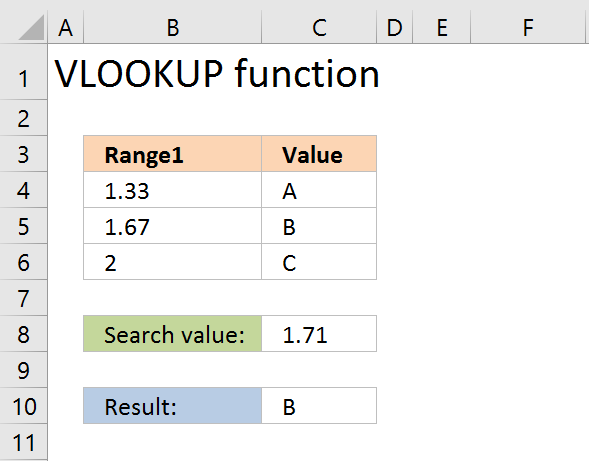=VLOOKUP(\$D\$8,\$B\$4:\$D\$6,3,TRUE)

The VLOOKUP function requires the table to be sorted based on range1 in an ascending order.

#### Explaining the VLOOKUP formula in cell C10

The VLOOKUP function looks for a value in the leftmost column of a table and then returns a value in the same row from a column you specify.

Arguments:
VLOOKUP(
lookup_value,
table_array,
col_index_num, [range_lookup]
)

The [range_lookup] argument is important in this case, it determines how the VLOOKUP function matches the lookup_value in the table_array.

The [range_lookup] is optional, it is either TRUE (default) or FALSE. It must be TRUE in our example here so that VLOOKUP returns an approximate match.

In order to do an approximate match the table_array must be sorted in an ascending order based on the first column.

=VLOOKUP(\$D\$8,\$B\$4:\$D\$6,3,TRUE)

becomes

=VLOOKUP(1,78,{1,33, 1,66, "A";1,67, 1,99, "B";2, 2,33, "C"},3,TRUE)

1,67 is the next largest value and the VLOOKUP function returns "B".

### INDEX + MATCH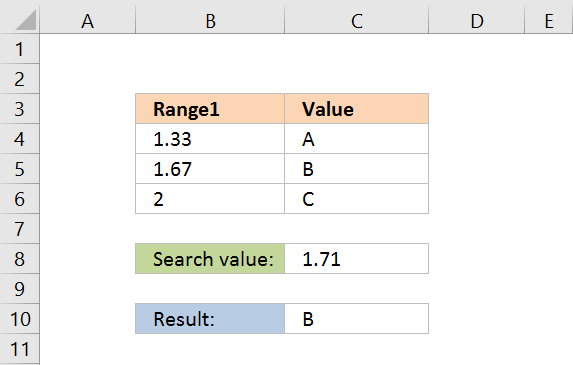Formula in cell C10:

=INDEX(\$D\$4:\$D\$6,MATCH(D8,\$B\$4:\$B\$6,1))

The lookup range must be sorted, just like the LOOKUP and VLOOKUP functions. Functions in this formula: INDEX and MATCH

Thanks JP!

#### Explaining INDEX+MATCH in cell D10

=INDEX(\$D\$4:\$D\$6,MATCH(D8,\$B\$4:\$B\$6,1))

Step 1 - Return the relative position of an item in an array

The MATCH function returns the relative position of an item in an array or cell range that matches a specified value

Arguments:
MATCH(
lookup_value,
lookup_array,
[match_type])
)

The [match_type] argument is optional. It can be either -1, 0, or 1. 1 is default value if omitted.

The match_type argument determines how the MATCH function matches the lookup_value with values in lookup_array.

We want it to do an approximate search so I am going to use 1 as the argument.

This will make the MATCH find the largest value that is less than or equal to lookup_value. However, the values in the lookup_array argument must be sorted in an ascending order.

=INDEX(\$D\$4:\$D\$6,MATCH(D8,\$B\$4:\$B\$6,1))

MATCH(D8,\$B\$4:\$B\$6,1)

becomes

MATCH(1.78,{1.33;1.67;2},1)

1.67 is the largest value that is less than or equal to lookup_value. 1.67 is the second value in the array. MATCH function returns 2.

Step 2 - Return a value of the cell at the intersection of a particular row and column

=INDEX(\$D\$4:\$D\$6,MATCH(D8,\$B\$4:\$B\$6,1))

becomes

=INDEX(\$D\$4:\$D\$6,2)

becomes

=INDEX({"A";"B";"C"},2)

and returns "B".

### Get the Excel fileReturn-value-if-in-rangev3.xlsx